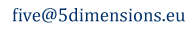# Serapioni - Real Masses  and Dark Matter

 “There remains one especially unsatisfactory feature [of the Standard Model of particle physics]: the observed masses of the particles, m. There is no theory that adequately explains these numbers. We use the numbers in all our theories, but we do not understand them – what they are, or where they come from. I believe that from a fundamental point of view, this is a very interesting and serious problem.” R.P. Feynman

 ... Standard Theory, which today can explain many phenomena that we observe in Physics, has a serious shortcoming whereby a wide range of experimental data has to be input into it without the explanatory support of a theoretical calculation ...  In order to address the nature of physical quantities, we introduce Fantappič's continuous group, published in "memoria serie VIII‑vol.XVII‑fasc.5" in November 1954, where each rotational symmetry can occur around the 5 axes of the space in which it is immersed.  ... Gödel's theorems establish that if the laws of nature are truly coherent, as we believe they are, then they must have some form of ... internal formulation that is different from anything that is known to us today ...  ... in recent years a number of elegant and powerful mathematical structures have come to light for a new definition of the concepts of space and time and they all seem to concur on one point: the existence of an "underlying structure" from which all physical quantities derive ... the only problem is that the structures that have been considered up to now "only represent a portion of the space that must be taken into consideration".  For a few decades now, theoretical physicists have entertained the hope that the length of the order of 10-13 cm, which is usually called λ, plays the role of elementary length in the future development of the theory. Just like c is the highest possible velocity in the theory of relativity, and h is the smallest possible action in quantum theory, λ is destined to play the role of the shortest possible distance in the future theory of matter. It will be, so to speak, “the diameter of a mathematical point,” and it will make no sense to speak about distances smaller than that. This possibility is a very interesting and exciting dream, which will probably come true, but nobody knows, at present, just when. ".  G. Gamow

## FIVE-DIMENSIONAL SPACE

But how is it possible that a length of the order of magnitude of 10-13 cm, usually denoted by the symbol λ , among the infinite number of possibilities, is so important in a 5D model of the universe ?

The value of λ is of such great importance because of the fact that it can be derived theoretically on the basis of a thought experiment in Thermodynamics.

Indeed, if we take a monatomic gas of mass m=1 travelling at a velocity V1 ≃ C and, by using a perfect diffuser, slow it down to a velocity   V2 ≃ 0

then the gas will undergo an increase in enthalpy:

ΔHMAX  =  CP ΔTMAX  =  (V12 -V2 2 ) / 2

Ignoring all the other forms of energy...

From this equation we can now derive the maximum stagnation temperature of this ideal gas considering that

2CP = R(ℓ+2)

(R)   is the Universal Gas Constant
(ℓ)   s the degrees of freedom of an element of the gas
CP   is the specific heat of the gas

We have taken into consideration a monatomic gas such as hydrogen with the (very probable!) condition that the protons can be considered not to have any internal degrees of freedom, so that ℓ, in this case, should coincide with the dimensions of the space in which each element of this gas can move.

Therefore, assuming ℓ = 5 we can calculate: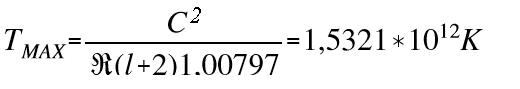Which shows that the maximum temperature Tº that can be obtained from this experiment agrees perfectly with the values of maximum temperature measured in real experiments when a ray of protons travelling at a velocity V2 ≃ c   collides with nearly stationary protons.

The theoretically calculated temperature however is only equal to the temperature measured experimentally (derived from the work of R, Hagedorm at the CERN in Geneva in 1971) if the number of degrees of freedom RR of the protons taken into account is five.

From this experiment we can deduce that  ℓ = 5 represents the effective dimensions of the physical space in which these particles move.

## The elementary length λ=λ0

The validity of the hypothesis that there exists a characteristic geometric distance λ=λ0 can also be confirmed by Thermodynamics, by calculating the maximum possible concentration of energy in a given point in space. Indeed, if there exists a maximum temperature TMAXthen there must also be a maximum energy

EMAX = κTMAX = 2,115250996 * 10-4 erg.

where κ = Boltzmann's constant

On the other hand, the maximum energy can also be defined with the equation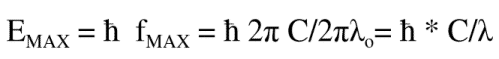where λ in this case is the minimum radius of an body's orbit in space, so that    fMAX = C/λ will be the maximum frequency that can exist at a point of a hypothetical five‑dimensional space.

Consequently, the maximum energy due to the maximum frequency that can exist in three‑dimensional space is:

EMAX" = 3/5 * ℏ * C/λ0

and equating   EMAX   with   EMAX "  we find that in three‑dimensional space there exists a minimum radius: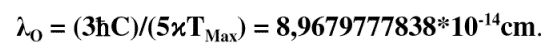The validity of this value can be confirmed by observing that it coincides perfectly with the value deduced from the classical electron radius and other combinations of fundamental constants like: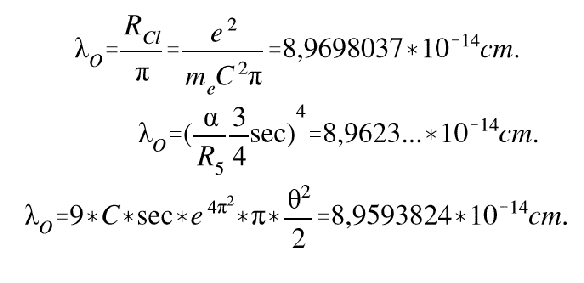where θ = 1,23....*10-21     α = fine-structure constant      R5 = 10 independent rotations in a 5D space

Coincident values that can not be attributed to chance

We shall make the hypothesis of making this fundamental characteristic geometric distance of physical space λ0, coincide with the time interval T =  [ λ01/4 ] .

In the following calculations therefore we shall use the fourth root of the modulus of the distance λ0 as a value for time T (or the minimum interval between two bodies in space-time)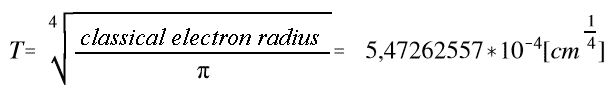as distance λ0 can indeed be considered the minimum interval between two points of a 5D space.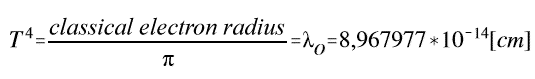## Fantappié's Continuous Group of Motions in 5D Space

We find that in this group the product of rotations of a modulus of time λ on 2D ST orbits, and on 3D XYZ volumes, coincide perfectly with the rotations on 2D, 3D and 4D surfaces in a 5D space.

2πλ0   *   4/3πλ03   =   8/3   *   π2 *   λ04

Rotations represented in the following picture:The originality of this λ0 motion does not only lie in the five dimensions, but in the assumption that, in addition to 3D XYZ space, it also rotates in the 2D ST dimensions.

It is therefore necessary to define the nature of this new ST space, insofar as, not being formed by the 3D XYZ dimensions of Eucleean geometry, it must correspond to unknown dimensions.

The value of all the real physical quantities prove to be definable by the kinetics of a modulus of distance λ0 that rotates in XYZST 5D space λ0 that rotates in XYZST 5D space.

Therefore when a particular radius R4 is inclined at an angle θ towards the radius in 3D, we find that the radius RST  =  R4  *  sinθ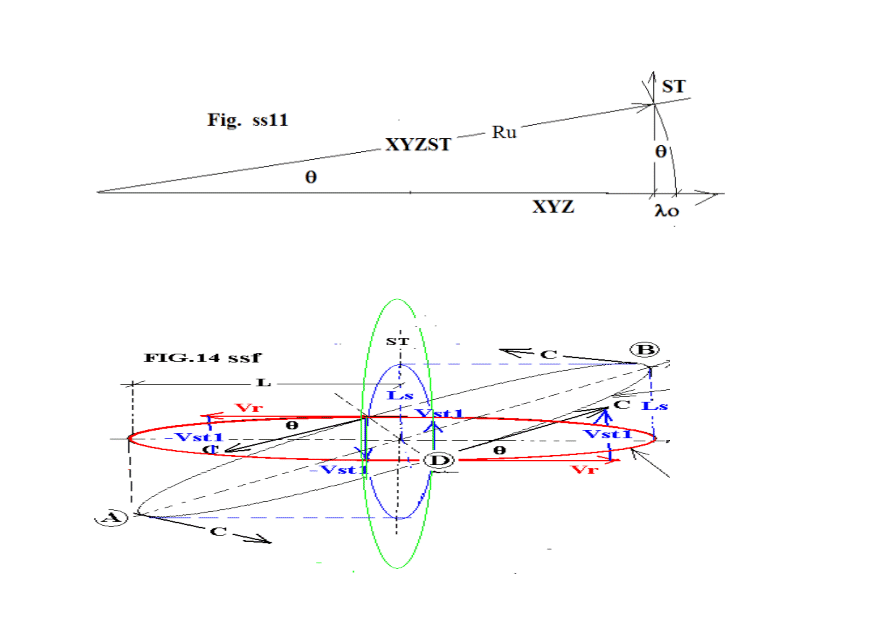We therefore find that when the radius R4 senθ coincides with the value of the normal radius RST in 2D, an angular velocity ωST is formed that is orthogonal to the angular velocity VXYZ in 3D space.

It will also transpire that when the dimensions of the radius RST = λX < λ0 , a radius of smaller dimensions than the fundamental radius   λ0 of real 5D space, is also defined.

And so if the radius λ0 gives rise to the minimum rotation of real quantities, then with  λX < λ0 no rotations can be made but only linear micro-displacements.

The existence of a new type of space is therefore found, that is only made up of micro-displacements  λX < λ0

Standard Theory considers Time to be made up of the Past and the Future
In 5D Space, Time is also considered to be made up of the Present and of Instants.

Let us define the "Present" as the period of time that is necessary to pass from the Past to the Future, by rotating with a radius  λ0  in a 4D orbit of 5D space.

On the other hand let us define an Instant Δt << Present to be a micro time interval that is not long enough for a radius λ0 to complete a full orbit, but only small linear displacements Δt  = λX < λ0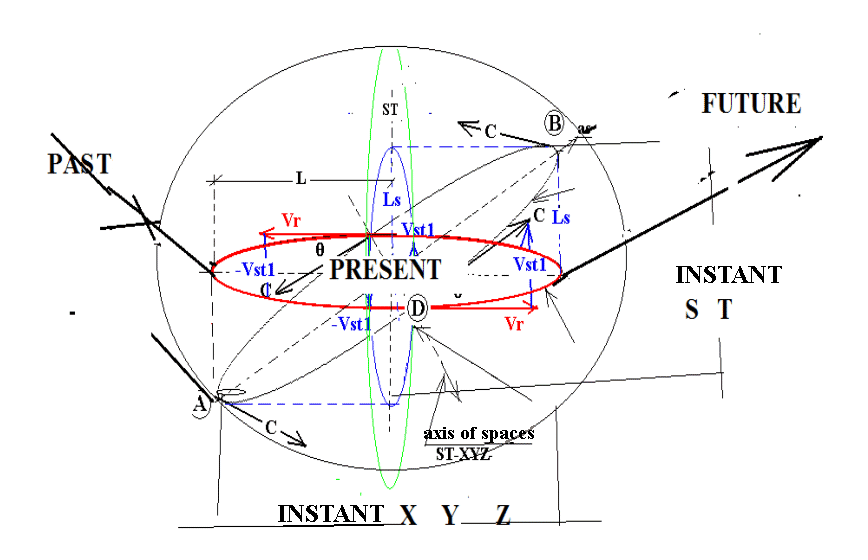And so in an instant Δt, it will be possible to effect instantaneous micro-displacements +- [ Δt..S.] +- [Δt.T..] in 2D ST space
and +- [Δt.X]+- [Δt.Y.] +- [Δt.Z.] in 3D XYZ space

And, therefore, there will only be linear micro-oscillations in 2D, 3D, and 4D spaces where λX < λ0 which will give rise to quantities ( λX λX3 λX4 ) in 5D space.
Five Dimensional space will therefore be made up of both
1)  rotations of real moduli λ0 in real space
2)  olinear oscillations of linear micro-moduli   Δtnbsp; =  λX < λ0 , hat do not belong to real space but a particular space underlying real space, let us call it underlying space (US).

It therefore transpires that motion in the Universe is made up of 2 types of space
A) one for the rotation of real radii λ0
B) and the other for very small linear displacements λX < λ0 that represent the instantaneous fundamental quantities Δt of an underlying structure (US).

MODULUS   Δt  =  λX

VELOCITY   Δt3  =  λX3

DISTANCE   Δt4  =  λX4

## Instantaneous quantities in Underlying Space (US)

A fundamental characteristic of 2D and 3D instantaneous micro displacements  is that in the time interval Δt invisible displacements λX of bodies are formed (only real λ0 quantities are considered visible).

Indeed, in an instant in 5D space only (λX0):

10 instantaneous micro-displacements λX in the invisible 2D ST planes

10 instantaneous micro-displacements λX in the invisible 3D X YZ volumes

can be formed, which will give rise to

10 quantities ΔS * Δt * Δt = [Δt3] in  ST spaces that we shall define as 10 invisible velocity vectors V [Δt3]

10 quantities ΔX * ΔY * ΔZ * Δt = [Δt4] n XYZ spaces that we shall define as 10 invisible distance vectors L [Δt4]

Ultimately we can conclude that in each instant Δt, quantities of velocity and distance, i.e. invisible velocity vectors and distance vectors L, are formed and subsequently cancelled in 5D (formed by 70 different instantaneous micro-displacements Δt ) which, due to the distance being λX < λ0 , will not form or emit energy.

Let us call these invisible quantities dark quantities.

Therefore, in the time interval [Δt1] 10 different velocity vectors Vst [Δt3] as well as 10 different distance vectors Lxyz [Δt4] can form, the product of which in turn can form 10 different instantaneous rotations

Vst [Δt3] ∧ LXYZ [Δt4] = [Δt7]

which "in turn”will form 5 invisible scalar quantities that we shall call Dark Masses

{ [Δt7] * [Δt7] } = [Δt14]

We therefore find that in an instant Δt, in the space underlying 5D space:

10 Instantaneous velocities V [Δt7]

10 Instantaneous distances L [Δt4]

10 Instantaneous rotations  V [Δt3] ∧ L [Δt4] = [Δt7] ,

can be formed together with 5 instantaneous scalar quantities [Δt14]

(  Velocity  [Δt3] ∧ Distance [Δt4] ) * ( Velocity [Δt3] ∧ Distance [Δt4] ) = [Δt14]

which represent 5 Instantaneous or Dark masses formed by invisible instants λX
We will see later that in order to form the value of real fundamental quantities of 5D space such as the age of the universe or the mass of the universe, it will also be necessary to calculate their entropy, which is the amount of energy that is necessary to create an order or a symmetry for these quantities which they apparently lack.
It will therefore always be necessary to use energy to form an ordered quantity starting from disordered elements.
And so in order to transform the group of 10 Velocity vectors [Δt3] = 30 [Δt]  and 10  distance vectors [Δt4] = 40 [Δt] ,  which create a set of 70 different instantaneous quantities [Δt] , energy will be required to build invisible ordered and symmetrical quantities like the masses of real quantities.

## Polar vectors

In XYZ space, where rotations can be right-handed or left-handed, the result of a right-handed rotation minus a left-handed rotation is null. In XYZS space certain rotations enable us to define these new quantities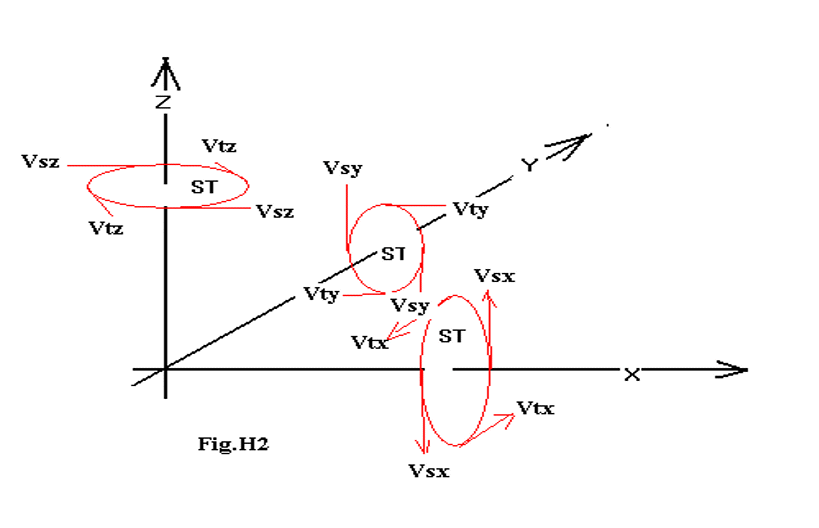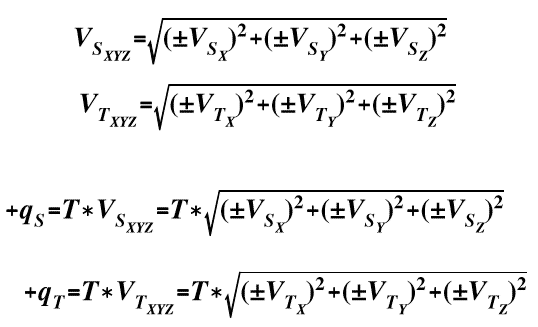where it appears that in this 5D space it is possible to define various types of velocities.

The number of quantities VSXYZ and VTXYZ , normal to XYZ, that can be defined with the VST(xyz) that exist in the XYZ system will therefore be 9, but as other different paths with velocity VXZ -Y -Z    VXY(Z-S-T)  etc. will also exist.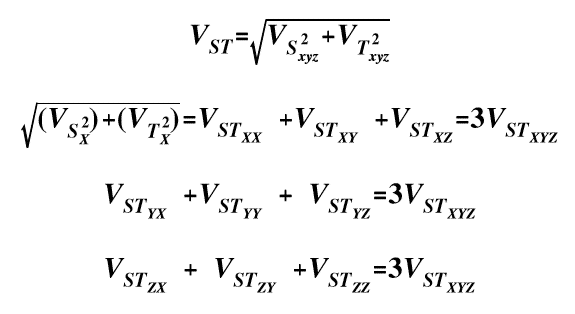we will find that out of these 9 types of velocity in 5D space there exist 10, like the

XY, XZ, XS, XT, YZ, YS, YT, ZS, ZT, ST planes

This will establish that a fundamental characteristic of 5D space is that of having bodies that can rotate with 90 velocities in different directions.

A body in the Present can therefore pass from the Past to the Future in 90 different directions.

The number 90 is one of the characteristics of 5D space that has links with Dirac and Euler.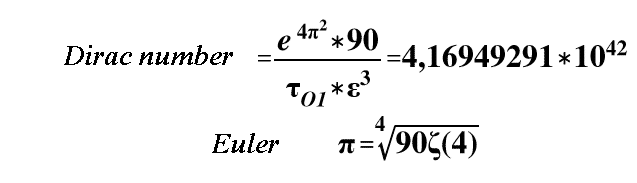5D space, where velocities VSxyz   VTxyz,) exist, can therefore generate 90 +qS and +qT quantities that are always positive and different.

## Dark Energy

Therefore, if 70 different instantaneous displacements are formed in an instant in 5D, energy will be necessary to transform them into ordered quantities, i.e. real quantities.

As a result, we can conclude that in 5D space, in order to obtain real energy, we must first transform them into 5 ordered real masses.

The value of Dark Masses, M O= 8.45*1056 gm, is 5 times greater than the real maximum mass which, in turn, is made up of a sum of randomly positioned masses.

And so in an Instant in 5D space, 5 disordered Dark masses are formed the value of which is 5 times the normal disordered mass that is determined as the sum of galaxies without a specific order.
Summarizing therefore, DARK masses are formed as a result of the product of:
10 micro-quantities  ([Δt] * [Δt]T * [Δt]S = 10 velocity V [Δt]3, formed by different XY XZ XS XI YZ TS YT ZS ZT ST .

10 micro-quantities [Δt] * [Δt]X * [Δt]Y * [Δt]Z = distances [Δt]4 , that are formed in different XYZ XYS XYT YZS YZT STX STY STZ ZYS ZXT ,
that will form 10 rotations V [Δt]3 ∧ L [Δt4] [Δt7]  which in turn will form the 5 different scalar quantities
[Δt]14 = ( [Δt]4 ∧ [Δt]3 ) *  [Δt]4 ∧ [Δt]3 )
i.e. 5 instantaneous masses positioned in five 4D hyper-volumes XYZS, XYZT, YZST, ZSTX, XYST, of 5D space.

But the energy of the 5 non-ordered instantaneous masses[Δt]14 obtained by multiplying by C2 is different from that measured experimentally.

Indeed, it was found that the experimental ratio between
Dark Energy / Overall Energy =(0.683)
does not coincide with the values of Dark Energy of non-ordered masses.

We have already seen that in order to derive the value of real fundamental quantities of 5D space, such as the mass of the universe, the age of the universe etc., it was necessary to calculate their entropy, i.e. the amount of energy that is necessary to create the symmetries of these real quantities.
In order to find the value of the Dark Energy of these masses, characterized by 70 random directions, it will first be necessary to transform them into ordered quantities.
It will therefore be necessary to have an amount of energy (entropy) to form order in these masses.
For this purpose we shall use the entropic coefficient
( ε4 = γ = 1,010756..)
Then, subtracting a certain amount of energy, calculated by using the coefficient (γ.= 1,010756 , from the 5 instantaneous Dark micro-masses    ( [Δt]4 ∧ [Δt]3 ) * [Δt]4 ∧ [Δt]3 ) = 5 * [Δt]14 situated in the five 4D hyper-volumes  XYZS XYZT YZST ZSTX XYST of 5D space,  an operation that involves a reduction of the energy of these disordered quantities by a factor of /γ70 the real value of Dark Energy as modified by entropy using the constant ( ε4 = γ = 1,010756..) will turn out to be

M O * C270 = 3,2196 * 1077erg / 2.14 = 1,5044 * 1077erg

While the simple real disordered energy will become

M * C2 = 1.518.. * 1077erg / γ70 (= 2,14) = 0,70934 * 1077erg

The total energy of the Universe will then be calculated only with Visible Energy /γ70 + Dark Energy /γ70 =

Energy of the Universe
1,5044 * 1077erg + 0,70934 * 1077erg = 2,21374 * 1077erg

which can then be applied to the Dark Energy / Energy of the Universe ratio:

Dark Energy / Energy of the Universe
1,5044 * 1077erg / 2,21374 = 0, 67958
0,683 / 0, 67958 = 1, 0050

which differs from the experimental value by 0.5% ...!

## Real Motion in 5D Space

Such motion is formed by angular velocity ωXYZ  in XYZ 3D space and angular velocity ωST , the latter formed by the orthogonal displacements of R4 sen θ = λ0 and radius R2 .
The two angular velocities ωXYZ ⊥ ωST will therefore create a rotation of a body in real 5D space , a particular motion from which we can theoretically derive the T2/T1 rotation times of the 2 angular velocities ωXYZ ⊥ ωST  from which all the fundamental constants of real physics can be derived with the following equations: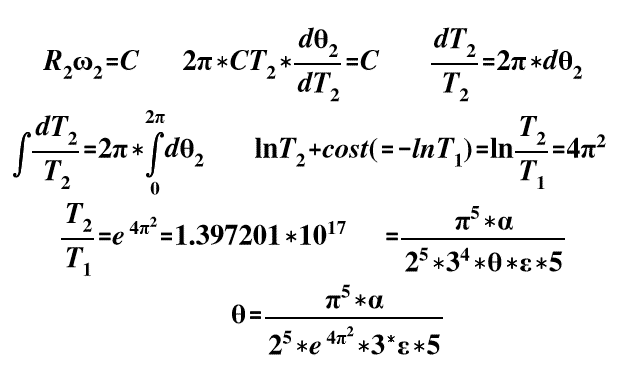Where, even taking into account Dirac's number: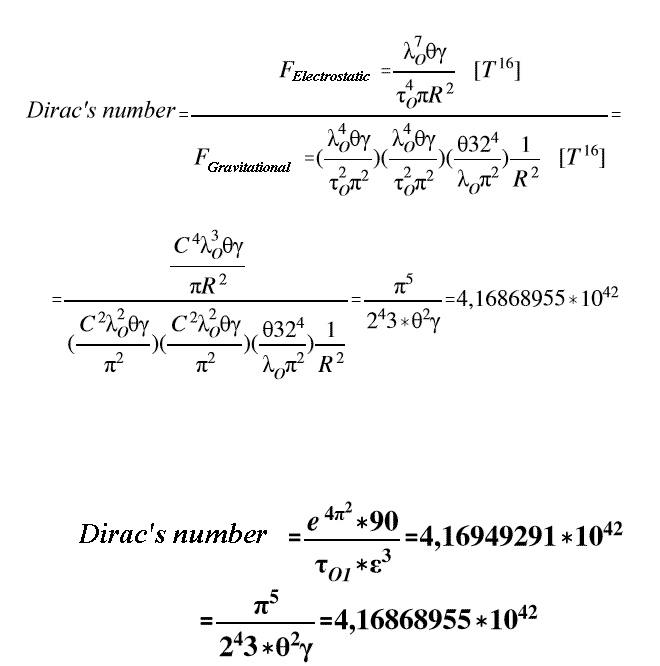it will be possible to deduce:
a)   the time for a T2/T1 rotation of a body that is rotating simultaneously in a 3D space and a 2D space
b)  the angle θ between 4D and 3D radii
c)  an equation containing 7 dimensionless constants with the compelling definitions of each physical quantity.

GF = ( 2∓A * 3∓B * 5 ∓C * π∓D ) * ( θ∓S * γ∓r * α∓0 * (T0N)

from which we deduce that in order to build kinematic motions that correspond to physical quantities we also need the specific constants γ (entropy) and α Heisenberg's constant.

All the experimental physical constants can therefore be derived theoretically with these coefficients that can only be deduced from a 5D space

speed of light c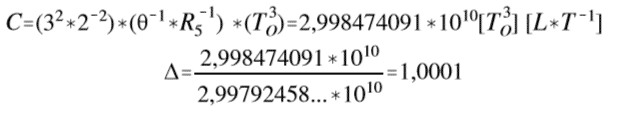gravitational constant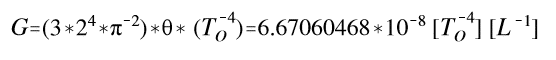electron mass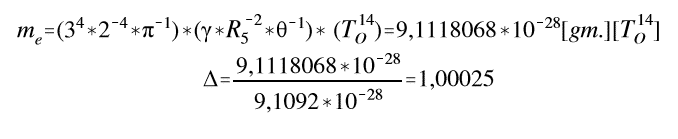proton massneutron mass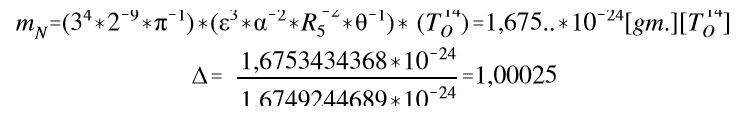Planck mass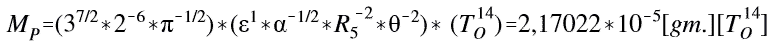elementary charge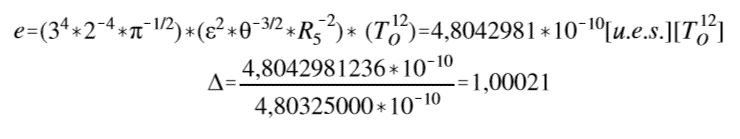Planck's constant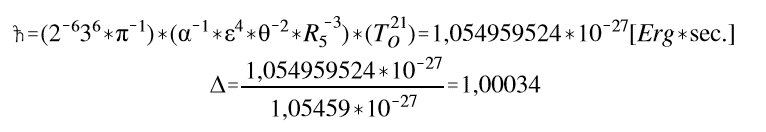fundamental length in String Theory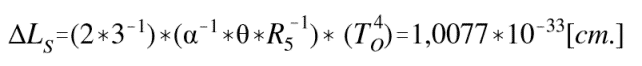## Other values for fundamental quantities theoretically derived from this model of 5D space

### Mass of the Universe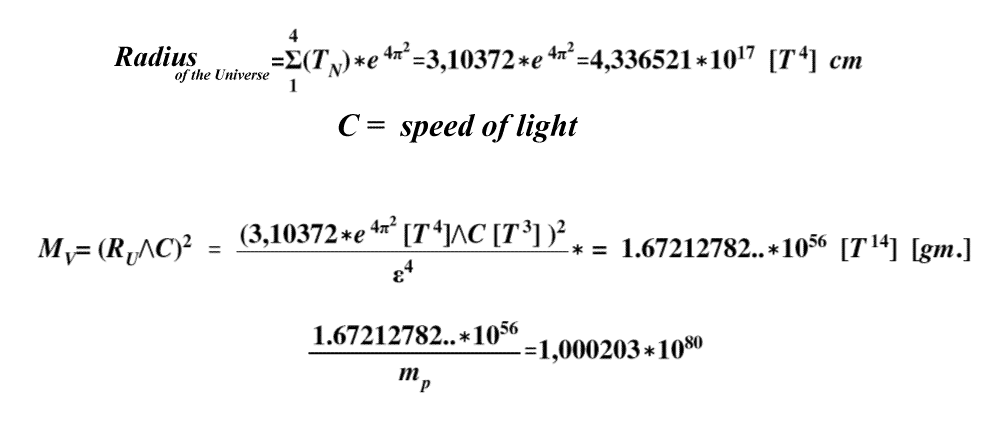### Age of the Universe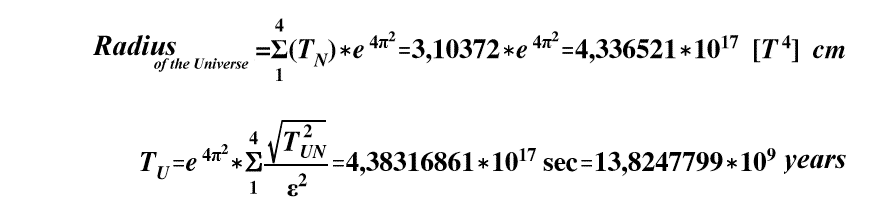The fundamental quantities of the universe coincide with the possibilities of a body to effect original rotations in 5D SS
Representing all the fundamental physical quantities by their rotation number tN, we will find that in 3D space the quantities

t1 = time period = Δ Ψ1
t3 = velocity V = Δ Ψ3 = Δ Ψ4 / Δ Ψ1
t4 = L = real distance = Δ Ψ4

are formed.

and in 5D space quantities are formed such as .....
t3 = surface area = L2 =L∧L....
t11 = Poynting vector = V∧L∧L
t12 = volume = L3 = L∧L∧L
t14 = M = mass = (V∧L) * (V∧L)
t16 = force = (V∧V∧V∧V∧L)
t17 = quantity of motion = [ (V∧L) * (V∧L) ] ∧V
t20 = energy = (V∧L)∧V * (V∧L)∧V
t21 = Planck's constant = Mom. Quant. Mot. (V∧L) * (V∧L) * (L∧V)

The number of fundamental orbits that are necessary to form all the fundamental physical quantities will therefore be

(1+3+4+8+11+12+14+16+17+20+21)=127

Moreover, due to their co-existence in the 10 planes of SS it will also be necessary to form N different orbits where

N = 5*10(1+3+4+8+11+12+14+16+17+20+21) = 5*10127

This coincidence shows that the masses, the energies etc. of the universe depend on groups of unique motions TN that can only exist in US with this number of orbits.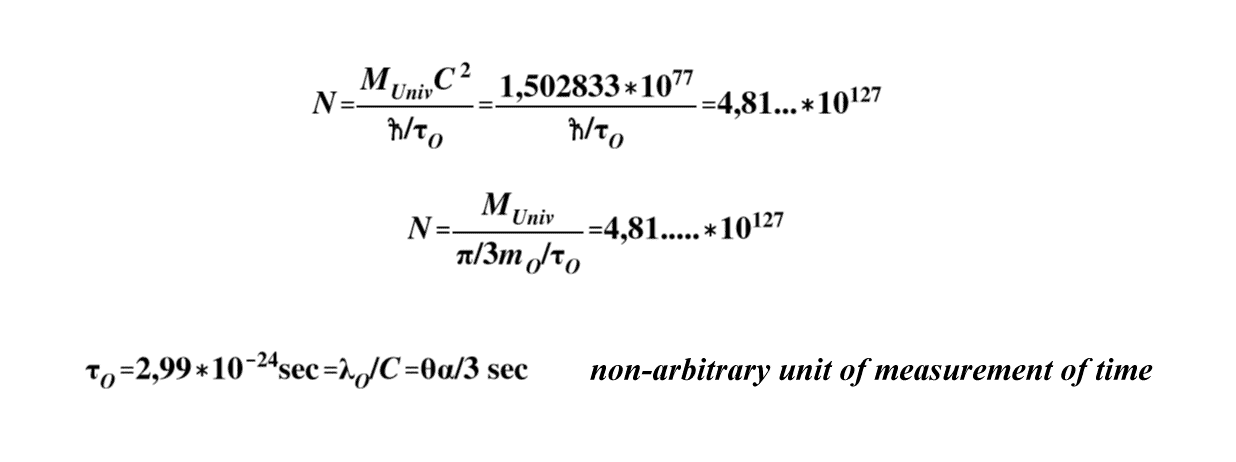And so all the orbits that are necessary to form existing fundamental physical quantities can be defined exclusively thanks to equation N. This is the number of different motions which are possible in the universe in every instant.

The French mathematician, physicist and engineer Gaspard-Gustave de Coriolis (1792-1843) identified the apparent acceleration of a body by observing its motion in a frame of reference that is rotating with respect to an inertial frame of reference...

Therefore, if we consider that in 5D space there are rotating micro-systems and linear micro-displacements, there will be micro-rotations in a 4D orbit, and linear micro-displacements in 2D space and in 3D space, that will define:

a) An acceleration to which a body is subjected with micro-rotations in a 5D orbit

b) An acceleration with instantaneous linear displacements in the XYZ and ST directions that form the acceleration to which a body in motion is subjected simultaneously in both a rotating and an inertial frame of reference …

... that will coincide with the acceleration that forms the rotation of bodies in 5D space.

## JULES-HENRY POINCARÉ'S CONJECTURE

A conjecture is an affirmation that is held to be true but that has not been formally and rigorously proved. The problem is all about understanding space, or rather spaces, from the point of view of their topological structure, that is to say the properties that remain invariant under continuous transformations.

It is all about classifying them in their various dimensions, identifying their characteristic properties and studying the link between these properties and the spaces of physical theories.
In Poincaré's original formulation, the conjecture states that every simply connected, closed 3‑manifold is (topologically) a 3‑sphere. A 3‑sphere in this case is the generalization of the usual sphere in three‑dimensional space (which is two‑dimensional and therefore a 2‑sphere).

In a less formal sense, the conjecture states that, in the same way as for a 2‑sphere, a 3‑sphere is the only possible type of closed three dimensional manifold "without any holes" (which reiterates the "simply connected" hypothesis)

This conjecture leads us to the question:

if an orbit in 4D that is formed by the product of instantaneous rotations of a 2‑sphere and a 3‑sphere that are simply connected, will the orbit on the 4D surface be also "simply connected"?

If we consider that in the case in which radii λX < λ0 represent non-curved quantities in a space with characteristics that are different from the characteristics of real space, then it should be possible to conjecture the existence of a product of instantaneous micro displacements in 2D and 3D that coincide with instantaneous micro displacements on a surface of a 4D space.
Will an instantaneous 4D surface formed by 2D surfaces be "simply connected"?
In a given instant, instantaneous 2D and 3D micro-rotations should form at point D on the surface of a 4D orbit. It would therefore appear that "simply connected" 4D instantaneous orbits can exist in a space where elements λX < λ0 exist.
And so in an instant ΔT, instantaneous micro-rotations should form in 2D ST space and in 3D XYZ space, specifically instantaneous micro rotations of 2πλx in 2D ST space and instantaneous rotations of 4/3 2 λx3 in 3D XYZ space, that coincide with instantaneous 8/3 * π2 * λx4 λx micro-rotations in a 4D orbit in 5D space.

BIG BANG DIMENSION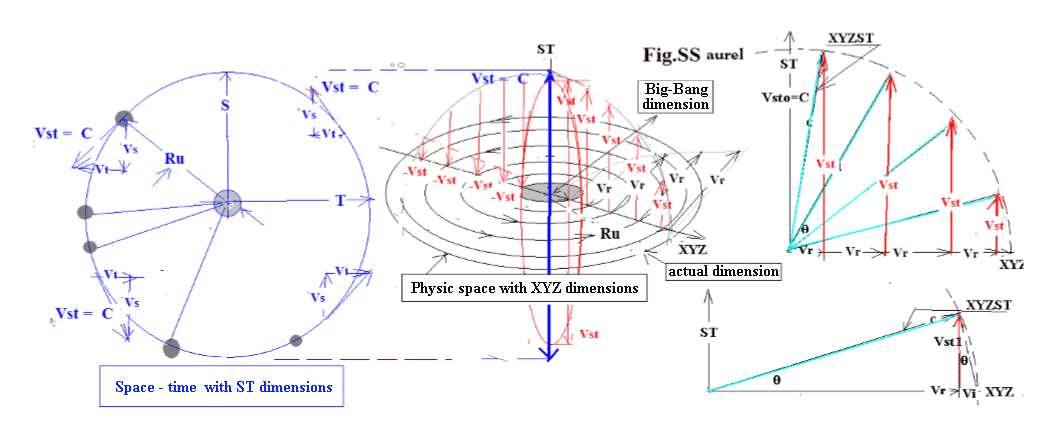Sergio Serapioni, 2016
Write to: